# HBSE 8th Class Maths Solutions Chapter 4 Practical Geometry Ex 4.5

Haryana State Board HBSE 8th Class Maths Solutions Chapter 4 Practical Geometry Ex 4.5 Textbook Exercise Questions and Answers.

## Haryana Board 8th Class Maths Solutions Chapter 4 Practical Geometry Exercise 4.5

Question 1.
Draw the following :
1. The square READ with RE = 5.1 cm.
2. A rhombus whose diagonals are 5.2 cm and 6.4 cm long.
3. A rectangle with adjacent sides of lengths 5 cm and 4 cm.
4. A parallelogram OKAY where OK = 5.5 cm and KA = 4.2 cm.
Solution:
(1) Rough Sketch: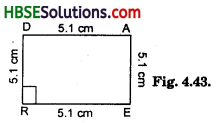Steps of Construction:
(i) Draw RE = 5.1 cm.
(ii) Make ∠YRE = 90° and ∠REX = 90°
(iii) Cut off DR = AE = 5.1 cm.
(iv) Join DA.
(v) DEAR is a required square.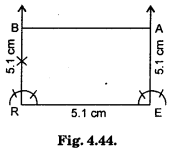(2) Rough Sketch: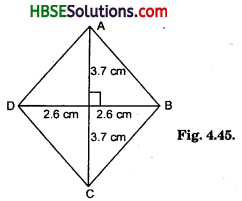Theory : Diagonals of a rhombus bisect each other at 90°
i.e., OA = OC = $$\frac{6.4}{2}$$ = 3.7 cm and OD = OB = $$\frac{5.2}{2}$$ = 2.6 cm
∠AOB = 90°
Steps of Construction :
(i) Draw BD = 5.2 cm.
(ii) Draw perpendicular bisector XY of BD.
(iii) Cut off OA = OC = 3.7 cm on the opposite side of DB.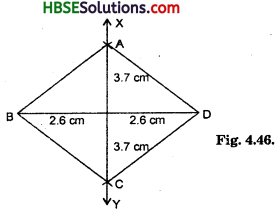(iv) Join AB, AD, CD and BC.
(v) ABCD is a required rhomus.(3) Rough Sketch :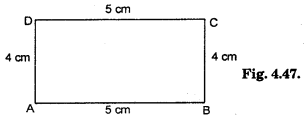Theory : A rectangle has equal opposite sides and each angles are 90°.
i.e. AB = DC = 5 cm DA = CB = 4 cm
∠A = ∠B = ∠C = ∠D = 90°
Steps of Construction :
(i) Draw a line segment AB = 5 cm.
(ii) Construct ∠A = ∠B = 90°.
(iii) Cut off AD = BC = 4 cm.
(iv) Join DC.
(v) You have a required rectangle ABCD.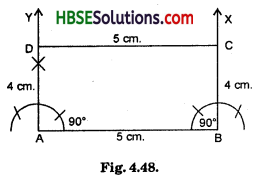4. Theory: Opposite sides of a parallelogram are equal and sum of a adjacent angle is 180°.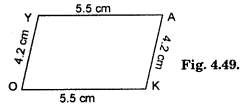i.e. OK = YA = 4.2 c.m. and OY = KA = 4.2 cm.
Steps of Construction :
(i) Draw OK = 5.5 cm
(ii) Let ∠K = 80°, so construct ∠OKX = 80°
(iii) Cut of FKA = 4.2 cm in KX.
(iv) Let the point be A.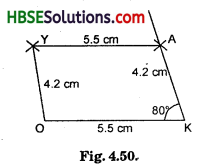(v) Draw arcs of 5.5 cm and 4.2 cm with centre as A and O respectively.
Note : You may take one angle of any measurement.